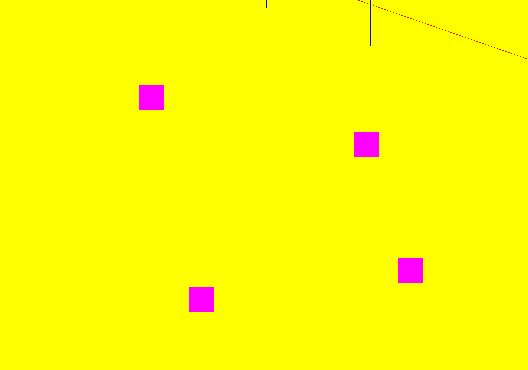# Weird World Coordinates/ Camera Problem

HI, I have been trying for a few days to sync screen coordinates to world coordinates, particularly the ground plane.

I have gotten some results
the points are plotted where the mouse points and that is greatuntil you zoom out and find them in a completely different location.

this is either a camera issue or a conversion issue…

conversion code(gathered from, a few sources):

``````def newPoint():
x, y = pygame.mouse.get_pos()

glEnable(GL_DEPTH_TEST)

depth = glReadPixels(x, y, 1, 1, GL_DEPTH_COMPONENT, GL_FLOAT)
print (depth)
model_view = np.array(glGetDoublev(GL_MODELVIEW_MATRIX))
proj = np.array(glGetDoublev(GL_PROJECTION_MATRIX))
view = np.array(glGetIntegerv(GL_VIEWPORT))
corrected_y = view - y-0.5
corrected_x = x+0.5

pointa = np.array(gluUnProject(x, corrected_y, depth, model_view, proj, view))
originalpt = np.array(gluUnProject(x, corrected_y, 0, model_view, proj, view))

print("pointa", pointa,"  originalpt",originalpt )
ray = pointa - originalpt
raydirection = ray/np.linalg.norm(ray)
print("ray", ray)
normal = np.array([0.0,1.0,0.0])

t = -((normal.dot(originalpt))/normal.dot(pointa))

point = originalpt+(t*raydirection)
print("tttt", t)
print(point,"point intrs" )
mylist.append(point)

``````

camera code(gathered from a few sources):

``````class createcamera:

def __init__(self, width, height):
self.viewport = width, height
self.viewport_aspect = self.viewport / self.viewport
eye_r = 20.0     # camera range, in meters
eye_th = 1   # camera azimuth angle, in radians
eye_phi = 1    # camera elevation angle, in radians

viewport = 1000, 1000
viewport_aspect = viewport / viewport

#how much of the world can we see, how wide is our sight
field_of_view = 45

lookat = (0,0,0)
location =np.array([eye_r*np.sin(eye_phi)*np.cos(eye_th),eye_r*np.cos(eye_phi),
eye_r*np.sin(eye_phi)*np.sin(eye_th)
])
camera_rotation = 0.0

near_plane = 0.1
far_plane = 50

def draw(self):
pass

def update(self):

glViewport(0, 0, self.viewport, self.viewport)
glMatrixMode(GL_PROJECTION)

gluPerspective(self.field_of_view,
1.0 * self.viewport / self.viewport,
self.near_plane, self.far_plane)

gluLookAt(self.eye_r*np.sin(self.eye_phi)*np.cos(self.eye_th),self.eye_r*np.cos(self.eye_phi),
self.eye_r*np.sin(self.eye_phi)*np.sin(self.eye_th),
self.lookat, self.lookat, self.lookat,
0.0, 1.0, 0.0)
print(self.location)
print(self.eye_r)

print ((self.location, self.location, self.location,
self.lookat, self.lookat, self.lookat,
0.0, 1.0, 0.0))
glMatrixMode(GL_MODELVIEW)

You need to fix the Y coordinate before using it to read from the depth buffer:

``````	depth = glReadPixels(x, view-1-y, 1, 1, GL_DEPTH_COMPONENT, GL_FLOAT)
``````

You need to find out why. The `glReadPixels` should occur after rendering; the depth buffer will be filled with 1.0 by `glClear`. Ensure that the viewport and matrices used for the `gluUnProject` call as the same as are used for rendering.